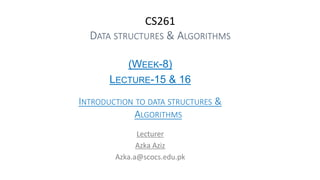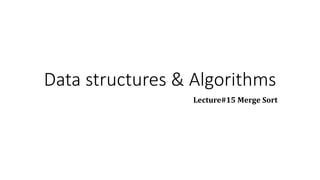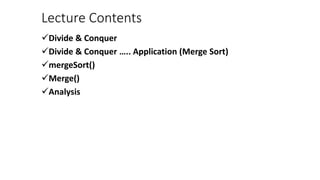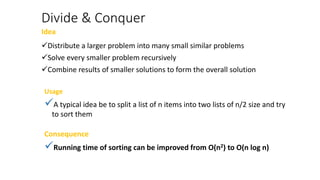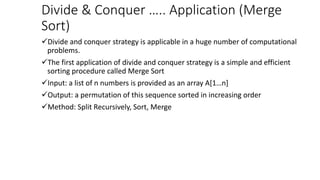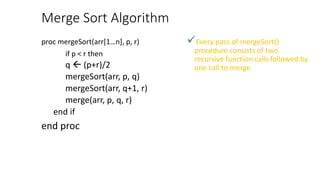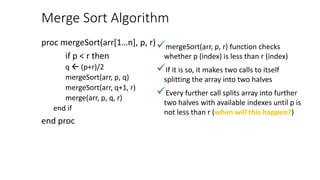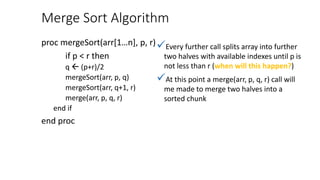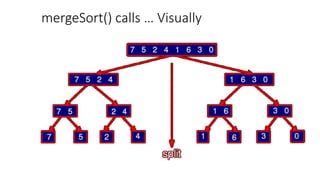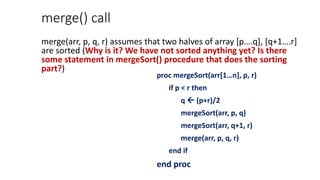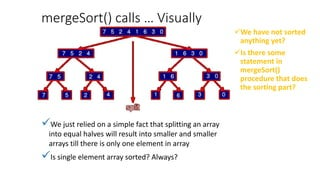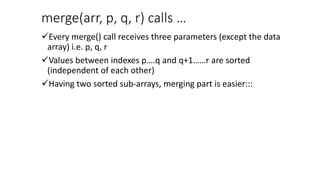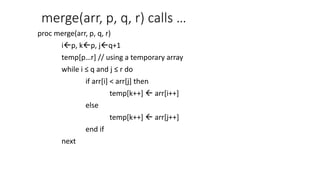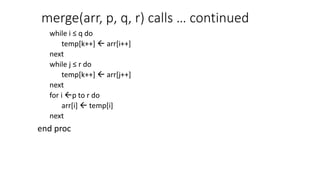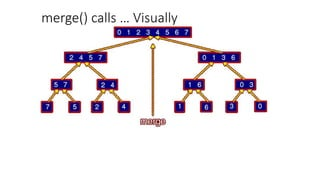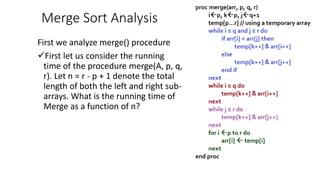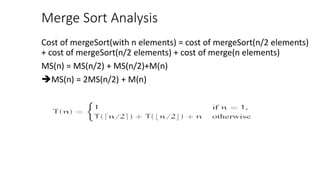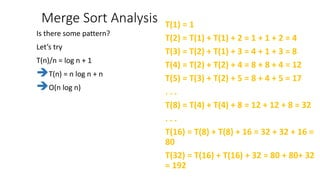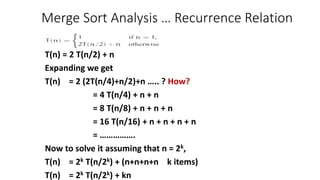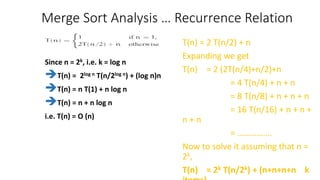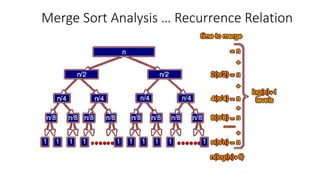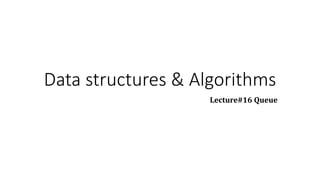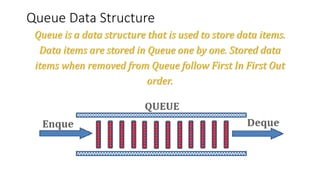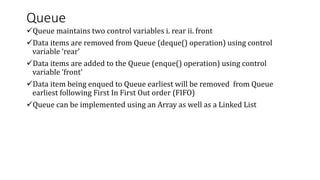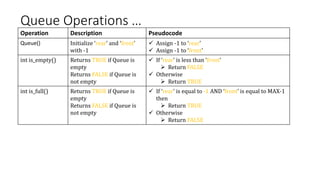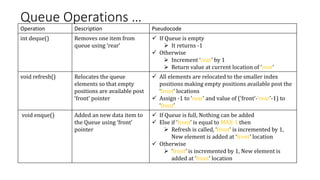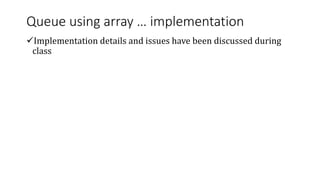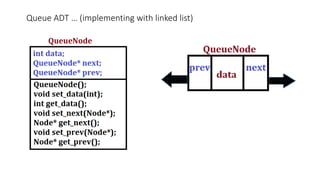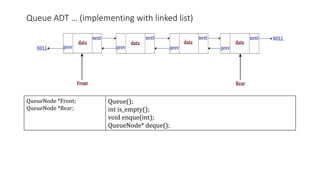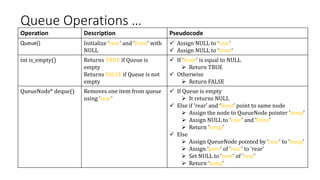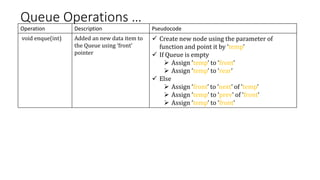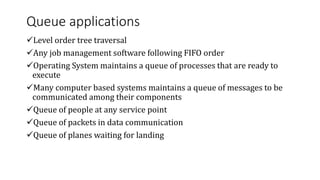1 of 34

### Data structure 8.pptx

1. CS261 DATA STRUCTURES & ALGORITHMS (WEEK-8) LECTURE-15 & 16 INTRODUCTION TO DATA STRUCTURES & ALGORITHMS Lecturer Azka Aziz Azka.a@scocs.edu.pk
2. Data structures & Algorithms Lecture#15 Merge Sort
3. Lecture Contents Divide & Conquer Divide & Conquer ….. Application (Merge Sort) mergeSort() Merge() Analysis
4. Divide & Conquer Idea Distribute a larger problem into many small similar problems Solve every smaller problem recursively Combine results of smaller solutions to form the overall solution Usage A typical idea be to split a list of n items into two lists of n/2 size and try to sort them Consequence Running time of sorting can be improved from O(n2) to O(n log n)
5. Divide & Conquer ….. Application (Merge Sort) Divide and conquer strategy is applicable in a huge number of computational problems. The first application of divide and conquer strategy is a simple and efficient sorting procedure called Merge Sort Input: a list of n numbers is provided as an array A[1…n] Output: a permutation of this sequence sorted in increasing order Method: Split Recursively, Sort, Merge
6. Merge Sort Algorithm proc mergeSort(arr[1…n], p, r) if p < r then q  (p+r)/2 mergeSort(arr, p, q) mergeSort(arr, q+1, r) merge(arr, p, q, r) end if end proc Every pass of mergeSort() procedure consists of two recursive function calls followed by one call to merge
7. Merge Sort Algorithm proc mergeSort(arr[1…n], p, r) if p < r then q  (p+r)/2 mergeSort(arr, p, q) mergeSort(arr, q+1, r) merge(arr, p, q, r) end if end proc mergeSort(arr, p, r) function checks whether p (index) is less than r (index) If it is so, it makes two calls to itself splitting the array into two halves Every further call splits array into further two halves with available indexes until p is not less than r (when will this happen?)
8. Merge Sort Algorithm proc mergeSort(arr[1…n], p, r) if p < r then q  (p+r)/2 mergeSort(arr, p, q) mergeSort(arr, q+1, r) merge(arr, p, q, r) end if end proc Every further call splits array into further two halves with available indexes until p is not less than r (when will this happen?) At this point a merge(arr, p, q, r) call will me made to merge two halves into a sorted chunk
9. mergeSort() calls … Visually
10. merge() call merge(arr, p, q, r) assumes that two halves of array [p….q], [q+1….r] are sorted (Why is it? We have not sorted anything yet? Is there some statement in mergeSort() procedure that does the sorting part?) proc mergeSort(arr[1…n], p, r) if p < r then q  (p+r)/2 mergeSort(arr, p, q) mergeSort(arr, q+1, r) merge(arr, p, q, r) end if end proc
11. mergeSort() calls … Visually We have not sorted anything yet? Is there some statement in mergeSort() procedure that does the sorting part? We just relied on a simple fact that splitting an array into equal halves will result into smaller and smaller arrays till there is only one element in array Is single element array sorted? Always?
12. merge(arr, p, q, r) calls … Every merge() call receives three parameters (except the data array) i.e. p, q, r Values between indexes p….q and q+1……r are sorted (independent of each other) Having two sorted sub-arrays, merging part is easier:::
13. merge(arr, p, q, r) calls … proc merge(arr, p, q, r) ip, kp, jq+1 temp[p…r] // using a temporary array while i ≤ q and j ≤ r do if arr[i] < arr[j] then temp[k++]  arr[i++] else temp[k++]  arr[j++] end if next
14. merge(arr, p, q, r) calls … continued while i ≤ q do temp[k++]  arr[i++] next while j ≤ r do temp[k++]  arr[j++] next for i p to r do arr[i]  temp[i] next end proc
15. merge() calls … Visually
16. Merge Sort Analysis First we analyze merge() procedure First let us consider the running time of the procedure merge(A, p, q, r). Let n = r - p + 1 denote the total length of both the left and right sub- arrays. What is the running time of Merge as a function of n?
17. Merge Sort Analysis Cost of mergeSort(with n elements) = cost of mergeSort(n/2 elements) + cost of mergeSort(n/2 elements) + cost of merge(n elements) MS(n) = MS(n/2) + MS(n/2)+M(n) MS(n) = 2MS(n/2) + M(n)
18. Merge Sort Analysis T(1) = 1 T(2) = T(1) + T(1) + 2 = 1 + 1 + 2 = 4 T(3) = T(2) + T(1) + 3 = 4 + 1 + 3 = 8 T(4) = T(2) + T(2) + 4 = 8 + 8 + 4 = 12 T(5) = T(3) + T(2) + 5 = 8 + 4 + 5 = 17 . . . T(8) = T(4) + T(4) + 8 = 12 + 12 + 8 = 32 . . . T(16) = T(8) + T(8) + 16 = 32 + 32 + 16 = 80 T(32) = T(16) + T(16) + 32 = 80 + 80+ 32 = 192
19. Merge Sort Analysis T(1) = 1 T(2) = T(1) + T(1) + 2 = 1 + 1 + 2 = 4 T(3) = T(2) + T(1) + 3 = 4 + 1 + 3 = 8 T(4) = T(2) + T(2) + 4 = 8 + 8 + 4 = 12 T(5) = T(3) + T(2) + 5 = 8 + 4 + 5 = 17 . . . T(8) = T(4) + T(4) + 8 = 12 + 12 + 8 = 32 . . . T(16) = T(8) + T(8) + 16 = 32 + 32 + 16 = 80 T(32) = T(16) + T(16) + 32 = 80 + 80+ 32 = 192 Is there some pattern? Let’s try T(n)/n = log n + 1 T(n) = n log n + n O(n log n)
20. Merge Sort Analysis … Recurrence Relation T(n) = 2 T(n/2) + n Expanding we get T(n) = 2 (2T(n/4)+n/2)+n ….. ? How? = 4 T(n/4) + n + n = 8 T(n/8) + n + n + n = 16 T(n/16) + n + n + n + n = ……………. Now to solve it assuming that n = 2k, T(n) = 2k T(n/2k) + (n+n+n+n k items) T(n) = 2k T(n/2k) + kn
21. Merge Sort Analysis … Recurrence Relation T(n) = 2 T(n/2) + n Expanding we get T(n) = 2 (2T(n/4)+n/2)+n = 4 T(n/4) + n + n = 8 T(n/8) + n + n + n = 16 T(n/16) + n + n + n + n = ……………. Now to solve it assuming that n = 2k, T(n) = 2k T(n/2k) + (n+n+n+n k Since n = 2k, i.e. k = log n T(n) = 2log n T(n/2log n) + (log n)n T(n) = n T(1) + n log n T(n) = n + n log n i.e. T(n) = O (n)
22. Merge Sort Analysis … Recurrence Relation
23. Data structures & Algorithms Lecture#16 Queue
24. Queue Data Structure Queue is a data structure that is used to store data items. Data items are stored in Queue one by one. Stored data items when removed from Queue follow First In First Out order.
25. Queue Queue maintains two control variables i. rear ii. front Data items are removed from Queue (deque() operation) using control variable ‘rear’ Data items are added to the Queue (enque() operation) using control variable ‘front’ Data item being enqued to Queue earliest will be removed from Queue earliest following First In First Out order (FIFO) Queue can be implemented using an Array as well as a Linked List
26. Queue Operations … Operation Description Pseudocode Queue() Initialize ‘rear’ and ‘front’ with -1  Assign -1 to ‘rear’  Assign -1 to ‘front’ int is_empty() Returns TRUE if Queue is empty Returns FALSE if Queue is not empty  If ‘rear’ is less than ‘front’  Return FALSE  Otherwise  Return TRUE int is_full() Returns TRUE if Queue is empty Returns FALSE if Queue is not empty  If ‘rear’ is equal to -1 AND ‘front’ is equal to MAX-1 then  Return TRUE  Otherwise  Return FALSE
27. Queue Operations … Operation Description Pseudocode int deque() Removes one item from queue using ‘rear’  If Queue is empty  It returns -1  Otherwise  Increment ‘rear’ by 1  Return value at current location of ‘rear’ void refresh() Relocates the queue elements so that empty positions are available post ‘front’ pointer  All elements are relocated to the smaller index positions making empty positions available post the ‘front’ locations  Assign -1 to ‘rear’ and value of (‘front’-’rear’-1) to ‘front’ void enque() Added an new data item to the Queue using ‘front’ pointer  If Queue is full, Nothing can be added  Else if ‘front’ is equal to MAX-1 then  Refresh is called, ‘front’ is incremented by 1, New element is added at ‘front’ location  Otherwise  ‘front’ is incremented by 1, New element is added at ‘front’ location
28. Queue using array … implementation Implementation details and issues have been discussed during class